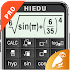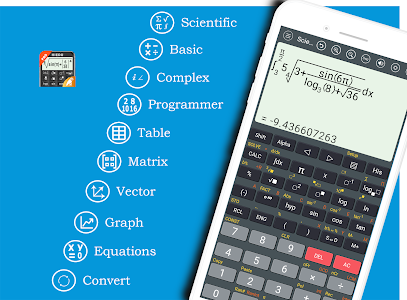# HiEdu Scientific Calculator Pro v1.0.8 (Paid) (SAP)

### HiEdu Scientific Calculator Pro / Screenshots### HiEdu Scientific Calculator Pro / Description

HiEdu Scientific Calculator Pro : A simple yet powerful calculator that includes standard scientific and programmer modes as well as a unit converter Math Physics and Chemistry Formulas graph and solve the equations . It's the perfect tool to add up a bill convert measurements in a recipe or other project and It is a very useful tool for education help complete complex math algebra or geometry problems.

KEY FEATURES
Scientific Calculator
• It is a scientific calculator supports most of the features of real calculators.
• Natural Display makes it possible to input and display fractions and certain functions (log x2 x3 x^ ) " x−1 10^ e^ d/dx Σ Abs ...) just as they are written in your textbook.
• Undo when you miss a mistake.
• Save history Select a calculation in list history and edit it.
• Create favorite calculations that make calculations faster.

Standard Calculator
• This is a perfect tool for daily calculations. With memory functions similar to a small handheld calculator
• Save history Select a calculation in list history and edit it.

Mathematics formulas
• This app has 1000+ math formula and more to come.
• Now no need to make paper notes to remember mathematics formulas just have this app put all the formulas on your favourite phones.
• you'll find formulas very simply explained in app with necessary figures will help you to understand very easily.

Physics formulas
• The application is a physical handbook. Contains most physical formulas for students and undergraduate.help users quickly refer to any Physics formulas for their study and work.This app displays most popular as well as advanced formulas in seven categories:
+ Mechanics
+ Electricity
+ Thermal physics
+ Periodic motion
+ Optics
+ Atomic physics
+ Constants

Chemical reactions
• Allows to discover chemical reactions and to solve the chemical equations with one and several unknown variables.

Programmers
• Can convert numbers between different number bases (2/8/10/16).
• Display shows numbers in binary hexadecimal octal and decimal.
• Input can be in binary hexadecimal octal or decimal.

Graphing calculator
• Easily graph functions solve equations find special points of functions.

Solve the equations
• Linear equation for degree onequadratic equation for degree twocubic equation for degree three System of linear equations.

Converter
• Available unit conversions include:
+ Currency (US dollar CDN dollar pound peso etc)
+ Temperature (celsius fahrenheit kelvin etc)
+ Length (kilometer miles meter yard feet etc)
+ Mass/Weight (kilogram pound ounce ton stone etc)
+ Speed (km/h mph knot etc)
+ Area (square kilometer square mile hectare acre etc)
+ Cooking Volume (teaspoon tablespoon cup pint quart ounce etc)
+ Pressure (kilopascal bar PSI etc)
+ Power (watt kilowatt horsepower etc)
+ Energy (joule calorie BTU etc)
+ Time (year month day hour second etc)
+ Fuel Consumption (miles per gallon liters per 100km etc)
+ Digital Storage (bit byte megabytes gigabytes etc)

DISPLAY
+ The layout of the buttons in the this app is scientifically rigorous which makes entering mathematical operations as simple and convenient as possible.
+ Numeric buttons and functions in the our application are beautifully designed clear resistant to glare blurry and eyestrain.
+ There are many beautiful themes with different styles.

### HiEdu Scientific Calculator Pro / What's New in v1.0.8 (Paid) (SAP)

- MOD INFO
Standalone Android Package
Android App Bundle Repacked (Split APKs Packer v6.7.1 by KirIif')

@srajawwal09

+) Add Accessibility, swipe on keyboard to click "Shift" , click "Alpha", to Back.

### Choose Download Locations for HiEdu Scientific Calculator Pro v1.0.8 (Paid) (SAP)

Upload files & Earn Huge Money
SignUp Now